# Bubble sort in Java - program to sort integer array

Bubble sort is one of the classic sorting algorithm which is used to explain sorting during various computer and engineering courses. Because of its algorithmic nature and simplicity its often used in various Java and C++ programming exercises. You may expect questions like Write Java program to sort integer array using bubble sort during any programming interview. Since algorithmic questions are always tricky question and not easy to code. Even simplest of them can lead to confusion, especially if you are not gifted with a natural programming head. I have seen many developers fumble if asked to code on the spot. That's why its advisable to do algorithmic and logical programming during training and learning programming and OOPS to get this skill of converting logic into code. Let's come back to Bubble sort, In Bubble sort algorithm we sort an unsorted array by starting from first element and comparing with adjacent element. If former is greater than later then we swap and by doing this we get the largest number at the end after first iteration. So in order to sort n elements you require n-1 iteration and almost n-1 comparison. For recap here is the logic for bubble sort sorting algorithm :

1) start comparing a to a
2) if a > a then swap numbers e.g. a=a and a=a
3) compare a to a and repeat till you compare last pair
4) This is referred as one pass and at the end of first pass largest number is at last
5) repeat this comparison again starting from a but this time going till second last pair only

Now let's see Java program which implements this bubble sort logic to sort unsorted integer array.

## How to sort integer array using bubble sort in JavaHere is complete code example of bubble sort in Java. It uses same algorithm as explained in first pass, it uses two loops. Inner loop is used to compare adjacent elements and outer loop is used to perform Iteration. because of using two loops it result in order of n^2 which is not great in terms of performance. If you are using Array List instead of array than you can sort them using Collections.sort method for better performance, for details check How to sort Array List in ascending and descending order.

package test;

import java.util.Arrays;

/**
* Java program to sort integer array using bubble sort sorting algorithm.
* bubble sort is one of the simplest sorting algorithm but performance
* of bubble sort is not good, its average and worst case performance
* ranges in O(n2) and that's why it is not used to sort large set of
* unsorted data. Bubble sort can be used for educational and testing
* purpose to sort small number of data to avoid performance penalty.
* This program is also a good example of how to print contents of Array in Java
*
* @author http://java67.blogspot.com
*/

public class BubbleSort {

public static void main(String args[]) {
//testing our bubble sort method in Java
int[] unsorted = {32, 39,21, 45, 23, 3};
bubbleSort(unsorted);

//one more testing of our bubble sort code logic in Java
int[] test = { 5, 3, 2, 1};
bubbleSort(test);

}

/*
* In bubble sort we need n-1 iteration to sort n elements
* at end of first iteration larget number is sorted and subsequently numbers smaller
* than that.
*/

public static void bubbleSort(int[] unsorted){
System.out.println("unsorted array before sorting : " + Arrays.toString(unsorted));

// Outer loop - need n-1 iteration to sort n elements
for(int i=0; i<unsorted.length -1; i++){

//Inner loop to perform comparision and swapping between adjacent numbers
//After each iteration one index from last is sorted
for(int j= 1; j<unsorted.length -i; j++){

//If current number is greater than swap those two
if(unsorted[j-1] > unsorted[j]){
int temp = unsorted[j];
unsorted[j] = unsorted[j-1];
unsorted[j-1] = temp;
}
}
System.out.printf("unsorted array after %d pass %s: %n", i+1, Arrays.toString(unsorted));
}
}

}

Output:
unsorted array before sorting : [32, 39, 21, 45, 23, 3]
unsorted array after 1 pass [32, 21, 39, 23, 3, 45]:
unsorted array after 2 pass [21, 32, 23, 3, 39, 45]:
unsorted array after 3 pass [21, 23, 3, 32, 39, 45]:
unsorted array after 4 pass [21, 3, 23, 32, 39, 45]:
unsorted array after 5 pass [3, 21, 23, 32, 39, 45]:
unsorted array before sorting : [5, 3, 2, 1]
unsorted array after 1 pass [3, 2, 1, 5]:
unsorted array after 2 pass [2, 1, 3, 5]:
unsorted array after 3 pass [1, 2, 3, 5]

That's all on How to sort integer array using Bubble sort in Java. We have seen a complete Java program for bubble sort and also printed output after each pass or iteration, if you look at carefully you will find that after each pass largest number gets sorted and number of comparison decreased. As I said Bubble sort is not a high performance sorting algorithm and you should by using Collection.sort() method from standard Java library to sort Collections or Arrays.sort() to sort Array in Java. Also this program demonstrate How to print contents of Array using Arrays.toString() as array in Java doesn’t override toString and simply printing array using System.out.println(array) will only show defulat toString from java.lang.Object class instead of contents of array.

Further Learning
The Coding Interview Bootcamp: Algorithms + Data Structures
Data Structures and Algorithms: Deep Dive Using Java
Algorithms and Data Structures - Part 1 and 2

Other programming tutorials from java67 you may find useful

1.I was looking for a Java program to implement Bubble sort algorithm, as part of my programming assignment. I just love the way you explain, its simply fantastic and explanation of Bubble sort algorithm itself is very self explanatory.

1.2.In Java, you can also sort array by using java.util.Arrays class as shown in this example

2.well done ...............

3.thanks for the explanation. it is really helpful

4.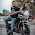how many iterations are involved in bubble sorting 5 integers

1.bubble sort is O(n^2) time complexity

5.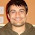Nice work Javin. Here is a more intuitive approach for implementing Bubble sort taken from original algorithm.

public static void bubblesort(int[] array) {
for (int i = 0; i < array.length; i++) {
for (int j = 0; j < array.length - 1 - i; j++) {
if (array[j] > array[j + 1]) {
int temp = array[j];
array[j] = array[j + 1];
array[j + 1] = temp;
}
}
}
}

6.Thanks Javin.
here may they ask to improve it performance or remove unwanted iteration, then you can simply add a variable to check is there any swaping is done for that main loop, if not break whole loop.
public static void bubbleSort(int[] unsorted){
System.out.println("unsorted array before sorting : " + Arrays.toString(unsorted));

// Outer loop - need n-1 iteration to sort n elements
for(int i=0; i unsorted[j]){
int temp = unsorted[j];
unsorted[j] = unsorted[j-1];
unsorted[j-1] = temp;
swap++;
}
}
if(swap==0)
break;
System.out.printf("unsorted array after %d pass %s: %n", i+1, Arrays.toString(unsorted));
}
}

7.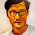This is not bubbling up the biggest --that is how bubble sort got the name .
This is the correct one:
int iarray[]....
int len=iarray.length
for (int end=len;end>0;end--)
for (int idx=0;idxiarray[iarray+1])
swap....

8.for the second iteration:
for(int j= 1; j<unsorted.length -i; j++)
it should be j<unsorted.length,
still need to compare to the last element.

1.we are comparing the last element by j+1.Therefore it should be j<unsortedlength-1-i or else it wil show OutOfBoundsException.

9.What if I am needing to use bubble sort on 50000 integers? Do I have to hard code all 50000 integers?

1.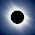Hello Paige, what do you mean by hard-coding here?

2.i think he means that he has to type all those 50000 integers but u can use .length for it

10.Really great nd helpful.

11.Thanks a lot

1.Glad to know Princy that you like this bubble sort program and my explanation.

12.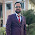The inner for loop which you are using for compare the element should be have condition like j < unsorted.length; not length-1.

13.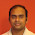@Javin, Does this looks okay, is there a problem using while loop with BubbleSort? I believe this would avoid unnecessary loops.

public static void bubbleSort(int arr[]){

int hold = 0;
boolean swapped = true;

while(swapped){
swapped = false;
for (int i : arr){
if(i < arr.length-1 && arr[i] > arr[i+1] ){
hold = arr[i+1];
arr[i+1] = arr[i];
arr[i] = hold;
swapped = true;

}
}
}

}

1.Yup, looks good to me but did you tested it for positive, negative and boundary conditions?